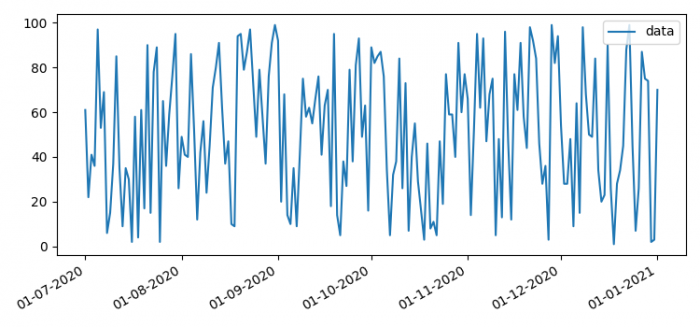# How to change the datetime tick label frequency for Matplotlib plots?

MatplotlibPythonData Visualization

To change the datetime tick label frequency for Matplotlib plots, we can create a dataframe and plot them in some date range

## Steps

• Set the figure size and adjust the padding between and around the subplots.
• To make potentially heterogeneous tabular data, use Pandas dataframe.
• Plot the dataframe using plot() method.
• Set X-axis major locator, i.e., ticks.
• Set X-axis major formatter, i.e., tick labels.
• Use autofmt_xdate(). Date ticklabels often overlap, so it is useful to rotate them and right align them.
• To display the figure, use show() method.

## Example

import pandas as pd
import numpy as np

import matplotlib.pyplot as plt
import matplotlib.dates as mdates

plt.rcParams["figure.figsize"] = [7.50, 3.50]
plt.rcParams["figure.autolayout"] = True

index = pd.date_range(start="2020-07-01", end="2021-01-01", freq="D")
index = [pd.to_datetime(date, format='%Y-%m-%d').date() for date in index]

data = np.random.randint(1, 100, size=len(index))

df = pd.DataFrame(data=data, index=index, columns=['data'])

ax = df.plot()
ax.xaxis.set_major_locator(mdates.MonthLocator(interval=1))
ax.xaxis.set_major_formatter(mdates.DateFormatter('%d-%m-%Y'))

plt.gcf().autofmt_xdate()

plt.show()

## Output